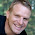First Law of Thermodynamics

In this, it states that both heat and work are mutually convertible. this is also said to be that when the heat is supplied to a system should equal to network done by it.
When there is both heat and work is transferred then the net energy stored in the system in form of internal energy.

It can be represented as,
Q-W=E
Where    Q = Heat is supplied to the system.
W= Work done by the system.
E= Change in internal energy.
In this process the energy is conserved. Energy is neither created nor be destroyed, it only converted from one form to another.

This leads us to know that the first law of thermodynamics is only another statement of the law of conservation.

1.